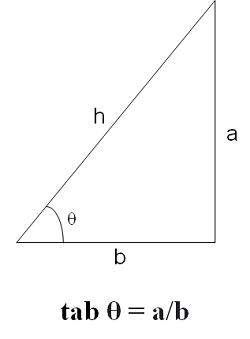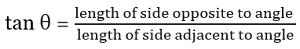# valarray tan() Function in C++ with Examples

C++ valarray tan() Function: Here, we will learn about the tan() function, its usages, syntax and examples.
Submitted by Shivang Yadav, on May 08, 2022

The valarray class in C++ is a special container that is used for holding elements like an array and performing operations on them.

tan function (mathematical Definition):

Mathematically, tangent is a trigonometric function that relate to the angle of a right-angled triangle. Its value is the ratio of the length of side opposite to the angle and side adjacent to the angle.## std::tan(std::valarray) Function

The tan() function of the valarray class is used to find the tangent value of each element of the valarray. The method calculates the tangent value and returns a new valarray consisting of the resultant value.

Syntax:

```template<class T> valarray<T> tan (const valarray<T>& x);

// or
tan(valarrayName)
```

Parameter(s): The method accepts a single parameter. It is the valarray on which the tan() function is applied.

Return Value: The method returns a valarray with an element whose value is equivalent to the cosine of the value of the original valarray.

## C++ valarray tan() Function Example 1:

```#include <iostream>
#include <valarray>
using namespace std;

int main()
{
// Declaring valarray
valarray<double> myvalarr = { 1, 0.2, 1.3, 2.4, 5 };

// Printing the elements of valarray
cout << "The elements of valarray are : ";
for (double& ele : myvalarr)
cout << ele << " ";

// Creating a new valarray of tan values
valarray<double> tanValarray;
tanValarray = tan(myvalarr);

cout << "\nThe elements of tan valarray is : ";
for (double& ele : tanValarray)
cout << ele << " ";

return 0;
}
```

Output:

```The elements of valarray are : 1 0.2 1.3 2.4 5
The elements of tan valarray is : 1.55741 0.20271 3.6021 -0.916014 -3.38052
```

The angles converted by the cosine function are taken in radians and the normal angle we read is in degree. Here, radian value is equivalent to the angles in degree.

```180° = π radians = 3.14159
90° = π/2 radians = 1.5708
60° = π/3 radians = 1.0472
45° = π/4 radians = 0.785398
30° = π/4 radians = 0.523599
```

Now, let's see the tan values of these.

## C++ valarray tan() Function Example 2:

```#include <iostream>
#include <valarray>
using namespace std;

int main()
{
// Declaring valarray
valarray<double> myvalarr = { 1, 0.2, 1.3, 2.4, 5 };

// Printing the elements of valarray
cout << "The elements of valarray are : ";
for (double& ele : myvalarr)
cout << ele << " ";

// Creating a new valarray of cos values
valarray<double> tanValarray;
tanValarray = tan(myvalarr);

cout << "\nThe elements of tan valarray is : ";
for (double& ele : tanValarray)
cout << ele << " ";

return 0;
}
```

Output:

```The elements of valarray are : 1 0.2 1.3 2.4 5
The elements of tan valarray is : 1.55741 0.20271 3.6021 -0.916014 -3.38052
```

Preparation